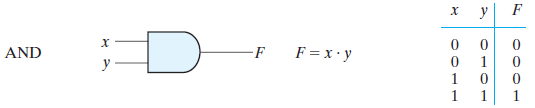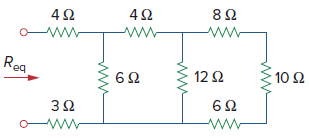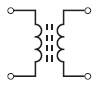# SSC JE Electrical Important MCQ Part 27

SSC JE Electrical Important MCQ PDF Part 27

1.Assume the series RLC circuit in Figure is at resonance.Then impedance Z isA. 500 Ω

B. 200 Ω

C. 100 Ω

D. 300 Ω

(XL equals XC at resonance; therefore XL=XC=500 Ω

Since the reactances cancel each other, the impedance equals the resistance.Zr = R = 100 Ω)

2.In the circuit of Fig. If K=2 Then value of current i isA. 4 A

B. -4 A

C. 0 A

D. 3 A

(Since 6 Ω and 3 Ω resistors are connected in parallel across the 24-V battery, i1 = 24/6 = 4 A. & i2=24/3=8 A

Applying KCL to node A, we get i − 4 + 4 K − 8 = 0 or i = 12 − 4 K.

When K = 2, i =12 − 4 K= 12 − 4 × 2 = 4 A)

3.Identify the fault shown in figureA. Single Line-to-Line Ground

B. Line – to – Line Fault

C. Double Line – to – line Ground Fault

D. Three-phase line to the ground fault

Answer : C (In double line-to-ground fault, the two lines come in contact with each other along with the ground. The probability of such types of faults is nearly 10 %.)

4.Transmission Lines shown in figure is aA. Pi Model of a Medium Transmission Line

B. T Model of a Medium Transmission Line

C. Short-Transmission Line

D. Long Transmission Line

Answer : A(Pi Model of a Medium Transmission Line-In nominal Pi model, it is assumed that the half of the capacitance concentrate at the each end of the line)

Electrical One Year Test Series Pass-Daily Test Plus Weekly Full Test

5.Circuit shown in figure is aA. Separately Excited DC Generator

B. Shunt Wound Generator

C. Series Wound Generator

D. Compound Wound Generator

Answer : A (A DC generators whose field winding or coil is energised by a separate or external DC source is called a separately excited DC Generator)

6.Given Truth Table forA.AND gate

B. OR gate

C. NOR gate

D. NAND gate7.Which pair of circuits in Fig. are equivalentA. a and b

B. b and d

C. a and c

D. c and d

Electrical One Year Test Series Pass-Daily Test Plus Weekly Full Test

(When the voltage source is connected with the resistance in series and it has to be converted into the current source than the resistance is connected in parallel with the current source a and c ,I=V/R=50/5=4A )

8. vo in the circuit of Fig isA. 20 V

B. 10 V

C. 30 V

D. 40 V9.The current i in the circuit of Fig. isA. −2.667 A

B.−0.667 A

C. 0.667 A

D. 2.667 A

(The loop equation for the circuit is

−10 + 4i + 6 + 2i = 0

6i=4

i=4/6=0.667 A)

10.Req in the circuit in Fig isA. 11 Ω

B. 12 Ω

C. 13 Ω

D. 14 Ω

(8Ω,10Ω and 6Ω in series =8+10+6=24 Ω

This 24 Ω in parallel with 12Ω=24×12/24+12=8Ω

This 8 Ω in series with 4Ω=8+4=12Ω

This 12 Ω in parallel with 6Ω=12×6/12+6=4Ω

This 4 Ω in series with 3Ω and 4Ω=4+3+4=11Ω)

11.A bridge circuit works at a frequency of 2 kHz. The following can be used as detectors for detection of null conditions in the bridge

C. Vibration galvanometers and tuneable amplifiers

D. Vibration galvanometer, headphones and tuneable amplifiers

(Detector- detector is a device which indicates zero deflection when the balance condition occurs.

Headphones-The headphones are used as detectors at the frequencies of 250 Hz to 3 to 4 kHz.

Vibration galvanometers -The vibration galvanometers are useful for low audio frequency range from 5 Hz to 1000
Hz but are commonly used below 200 Hz.

Tunable amplifier-Tunable amplifier detectors are used for frequency range of 10 Hz to 100 Hz.)

Electrical One Year Test Series Pass-Daily Test Plus Weekly Full Test

12.Wagner’s earth device is used in ac bridge circuits for

A. Eliminating the effect of earth capacitances

B. Eliminating the effect of inter-component capacitances

C. Eliminating the effect stray electrostatic fields

D. Shielding the bridge elements

Answer : A (The Wagner earthing device is used for removing the earth capacitance from the bridges.)

13.Maxwell inductance capacitance bridge is used for measurement of inductance of

A. Low Q coils

B. Medium Q coils

C. High Q coils

D. Low and medium Q coils

Answer : B (Maxwell inductance capacitance bridge-In Maxwell’s inductance capacitance bridge the value of inductance is measured by the comparison with standard variable capacitance. Maxwell Bridge is suitable for the measurement of inductance with medium vale of Q (1<Q<10).)

14.Units generated/annum is equal to

A. Max. demand (in kW) × L.F.

B. Max. demand (in kW) × L.F. × 8760

C. Average load (in kW) × L.F. × 8760

D. Average load (in kW) × L.F.

∴ Average load = Max. demand × L.F.

Units generated/annum = Average load (in kW) × Hours in a year

= Max. demand (in kW) × L.F. × 8760)

15.The ratio of kWh generated to the product of plant capacity and the number of hours for which the plant was in operation is known as

A. Plant use factor

B. Plant capacity factor

C. Diversity factor

(Plant use factor. It is ratio of kWh generated to the product of plant capacity and the number of hours for which the plant was in operation

Plant use factor = Station output in kWh Plant capacity × Hours of use)

16.The ratio of actual energy produced to the maximum possible energy that could have been produced during a given period is known as

A. Reserve capacity

B. Plant capacity factor

C. Diversity factor

(Plant capacity factor = Actual energy produced/Max. energy that could have been produced

=Average demand × T/Plant capacity × T

=Average demand/Plant capacity

Thus if the considered period is one year,Annual plant capacity factor =Annual kWh output/Plant capacity × 8760)

17.The ratio of average load to the maximum demand during a given period is known as

A. Demand factor

B. Diversity factor

C. Plant capacity factor

(The ratio of average load to the maximum demand during a given period is known as load factor

If the plant is in operation for T hours,Load factor = Average load × T/Max. demand × T

=Units generated in T hours/Max. demand × T hours

Load factor is always less than 1 because average load is smaller than the maximum demand.

Higher the load factor of the power station, lesser will be the cost per unit generated)

Electrical One Year Test Series Pass-Daily Test Plus Weekly Full Test

18.The ratio of maximum demand on the power station to its connected load is known as

A. Demand factor

C. Diversity factor

D. Plant capacity factor

(Demand factor. It is the ratio of maximum demand on the power station to its connected load

Demand factor = Maximum demand Connected load

The value of demand factor is usually less than 1.

It is expected because maximum demand on the power station is generally less than the connected load.)

19. The ratio of the sum of individual maximum demands to the maximum demand on power station is known as

A. Diversity factor

B. Plant capacity factor

D. Demand factor

(The ratio of the sum of individual maximum demands to the maximum demand on power station is known as diversity factor

Diversity factor = Sum of individual max. demands/Max. demand on power station

The maximum demand on the power station is always less than the sum of individual maximum demands of the consumers. Obviously, diversity factor will always be greater than 1.

The greater the diversity factor, the lesser is the cost of generation of power.)

20.Schematic circuit symbols shown in Figure is aA. Inductors

B. Speaker

C. Amplifier

D. Transformers

Answer : D (Schematic circuit symbols for a ferrite core transformers)